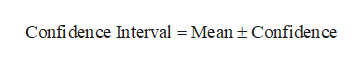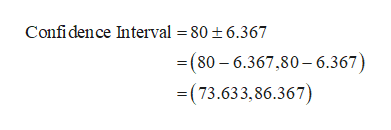1. In a random sample of 15 CD players brought in form repair, the average repair cost was \$80 and the sample standard deviation was \$14. Construct a 90% confidence interval for. Assume the repair costs are normally distributed and there are no outliers.

Question

1. In a random sample of 15 CD players brought in form repair, the average repair cost was \$80 and the sample standard deviation was \$14. Construct a 90% confidence interval for. Assume the repair costs are normally distributed and there are no outliers.

Step 1

It is given that the average repair cost (mean) is \$80, sample standard deviation s is \$14, the sample size (n ) is 15 and the level of confidence is 90%.

Step 2

The formula for finding the 90% confidence interval for mean is as follows:help_outlineImage TranscriptioncloseConfi dence Interval = Mean t Confidence fullscreen
Step 3

The confidence is 6.367 using Excel formula “=CONFIDENCE.T((1–0.90),14,15)”.

Substitut...help_outlineImage TranscriptioncloseConfidence Interval = 80 t6.367 (80 6.367,80-6.367) (73.633,86.367) fullscreen

Want to see the full answer?

See Solution

Want to see this answer and more?

Our solutions are written by experts, many with advanced degrees, and available 24/7

See Solution
Tagged in

Other